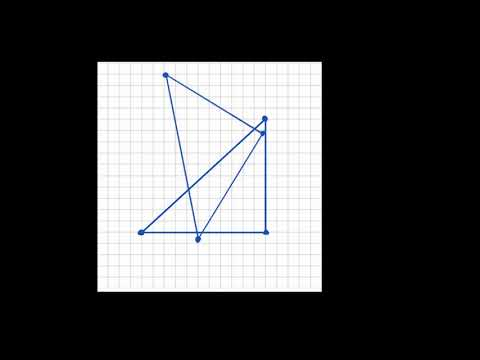# Angles in a triangle sum to 180° proof (Full video)

Description: Learn the formal proof that shows the measures of interior angles of a triangle sum to 180°. Created by Sal Khan. And what I want to prove is that the sum of the measures of the interior angles of a triangle, that x plus y plus z is equal to 180 degrees. And the way that I'm going to do it is using our knowledge of parallel lines, or transversals of parallel lines, and corresponding angles. And to do that, I'm going to extend each of these sides of the triangle, which right now are line segments, but extend them into lines.

### Other videos you might be interested in### Angles, parallel lines, & transversals (Full video)